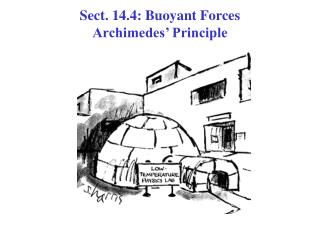# Sect. 14.4: Buoyant Forces Archimedes’ Principle - PowerPoint PPT PresentationDownload PresentationSect. 14.4: Buoyant Forces Archimedes’ Principle

Sect. 14.4: Buoyant Forces Archimedes’ Principle
Download Presentation## Sect. 14.4: Buoyant Forces Archimedes’ Principle

- - - - - - - - - - - - - - - - - - - - - - - - - - - E N D - - - - - - - - - - - - - - - - - - - - - - - - - - -
##### Presentation Transcript

1. Sect. 14.4: Buoyant Forces Archimedes’ Principle

2. Experimental facts: 1.Objects submerged (or partially submerged) in a fluid APPEARto “weigh” less than in air. 2.When placed in a fluid, many objects float! Both are examples ofBUOYANCY • See figure. An object submerged in astatic fluidexperiences an upwardBUOYANT FORCE Bas well as the downward gravitational force Fg • This Buoyant Force Bis exerted by a fluid on an immersed object. Since the fluid is static, the object doesn’t move. • So, the buoyant force balances the downward gravitational force, so that Newton’s 2nd Lawin the vertical direction ∑Fy = 0, is satisfied.

3. By Newton’s 2nd Law, ∑Fy = 0, the magnitude of the buoyant B force must be equal (in magnitude) to the downward gravitational force. Fg • B is the resultant force due to all forces applied by the fluid surrounding the object. • The person who first realized how to calculate B was an ancient Greek mathematician named Archimedes

4. Archimedes • C. 287 – 212 BC • Greek mathematician, physicist and engineer • First to calculate the ratio of a circle’s circumference to it’s diameter (π) • Calculated volumes of various shapes • Discovered the nature of the buoyant force • Inventor of many practical devices: • Catapults, levers, screws, etc.

5. Buoyant Force:Occurs becausethe pressure in a fluid increases with depth! P = ρg h (fluid atREST!!)

6. Experiments findthat the magnitude ofthe buoyant forceBis always equal to the weight of the fluid displaced by the object • This is called Archimedes’ Principle • Archimedes’s Principle does not refer to the makeup of the object experiencing the buoyant force • The object’s composition is not important since the buoyant force is exerted by the fluid

7. To understandArchimedes’ Principlein more detail, consider the simple case of a cubic shaped object(height h, cross sectional area A)floating submerged in a fluid of density ρfluid as in the figure. • Use the definition of pressure in terms of area & force: The pressure Ptop at the top of the cube causes a downward force Fdown = PtopA on the upper face. • The pressure Pbot at the bottom of the cube causes an upward force Fup = PbotA on the lower face. • Total force the fluid exerts on the cube:B = (Pbot – Ptop)A(1) • Use the dependence of pressure on depth:(Pbot – Ptop) = ρfluidgh(2) • Combining (1) & (2) gives B = ρfluidghA = ρfluidgV = Mg. Mg is the weight of the FLUID displaced by the object!

8. Archimedes Principle The total (upward) buoyant force Bon an objectof volume Vcompletely or partially submerged in a fluid with density ρfluid: B= ρfluidVg (1) ρfluidV  M Mass of fluid which would take up same volume as object, if object were not there. (Mass of fluid that used to be where object is!)  Upward buoyant force B= Mg (2) B= weight of fluid displaced by the object! (1) or (2) Archimedes Principle Proved for cylinder. Can show valid for any shape

9. Archimedes Principle B B B B B

10. Archimedes Principlesays that if an object of volume Vobj is totally submerged in a fluid of density ρfluid • The upward buoyant force is B = ρfluidgVobj • Of course, the downward gravitational force is Fg = Mg = ρobjgVobj • If the object is floating submerged & not moving, by Newton’s 2nd Lawin the vertical direction, ∑Fy = 0, the net vertical force must be zero: B - Fg = (ρfluid – ρobj)gVobj = 0

11. Of course, objects don’t always float motionless when submerged! From the previous slide, for this to happen, we must have: (ρfluid – ρobj)gVobj = 0 or, ρfluid = ρobj. That is, the object & the fluid must have the same density! • If the object’s density is less than the fluid density, (ρfluid > ρobj), by Newton’s 2nd Law, ∑Fy = Ma, the object accelerates upward, as in Fig. a • If the object’s density is greater than the fluid density, (ρfluid < ρobj), by Newton’s 2nd Law, ∑Fy = -Ma, the object accelerates downward (it sinks!), as in Fig. b. • So, Archimedes’ Principle tells us that: The direction of the motion of an object in a fluid is determined only by the densities of the fluid and the object

12. Archimedes Principle: Floating Objects Free body diagram  • Consider an object of volume Vobj& density ρobj floating (partially submerged) at top of a fluid of density ρfluid. See figure: • Object is not moving soNewton’s 2nd Lawin the vertical direction,∑Fy = 0, tells us that the upward buoyant force B is balanced by the downward force of gravity Fg. This gives: B - Fg = ρfluidgVfluid- ρobjgVobj= 0 or ρfluidVfluid= ρobjVobj • Here, the volume of the fluid displaced by the object, Vfluidisn’t the object volume but is the portion of the volume of the object beneath the fluid level. The last relation can be rewritten as: 

13. Archimedes Principle:Floating objects in equilibrium:  ∑Fy = B - Fg = 0 Gives: ρfluidVfluid= ρobjVobj or f = f Fraction of the volume of floating object which is submerged.  Archimedes’ Principle: The fraction of a floating object which is submerged is equal to the ratio of the density of the object to the density of the fluid.